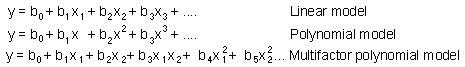4.1. Correlation between population density and factors

Statistical models are used to analyze the correlation between the population density and various factors that change in time and/or in space, e.g., temperature, precipitation, elevation, etc. These models can be used to predict population numbers in the future or in unsampled spatial locations.

Data may be of 3 types:

1. Time series - a sequence of measurements at the same point in space
2. Spatial series - a set of measurements made (simultaneously or not) at different points in space
3. Mixed series - a set of time series obtained from different points in space
Statistical models are usually represented by linear or polynomial equations:where y is the predicted variable (e.g., population density); Xi are factors; and bi are parameters which can be found using regression analysis.

Autoregressive model is a regression model in which the value of the dependent variable is predicted from values of the same variable in previous time steps or in neighboring locations in space. Most statistical models of population dynamics are autoregressive. For example, the density of the population in the next year can often be predicted from its current density and/or its density in 1 or 2 previous years. In spatio-temporal models, population density in a specific location in the next year can be predicted from the current density in the same location as well as in neighboring locations. Autoreggressive models may include external factors, i.e., climatic factors.

Geostatistics (kriging, see Lecture 2) is a better method for spatial modeling than standard regression. It is possible to use a 3-dimensional kriging (2 space coordinates, and 1 time coordinate) for spatio-temporal models .

The mechanisms that cause correlations between population density and factors are not represented in these models. Prediction is based solely on the previous behavior of the system. If in the past the system exhibited specific behavior in particular situations, then it is most probable that it behave in a similar way in the future. Statistical modeling is based on the assumption that system behavior remains the same. Thus, these models have very limited value for predicting new behaviors of the system. For example, if we develop a new method for controlling pest populations or a new strategy for conservation of some species, then stochastic models based on the previous dynamics of these populations may be misleading.

In some cases stochastic models may help to understand some mechanisms of population dynamics. For example, if insect population density increases in years with hot and dry summer, then it is possible that insects of this species have low mortality in these years, possibly due to reduced resistance of host plants. Of course, these hypotheses require thorough testing.

Alexei Sharov 2/03/97